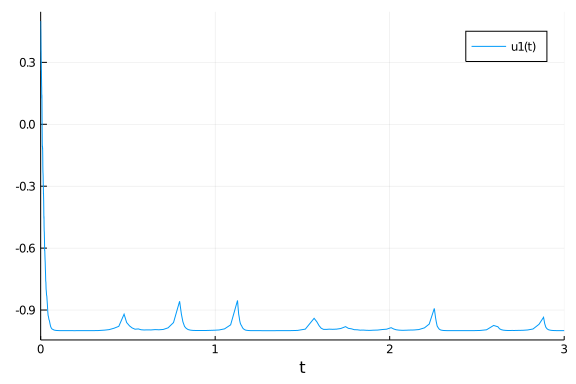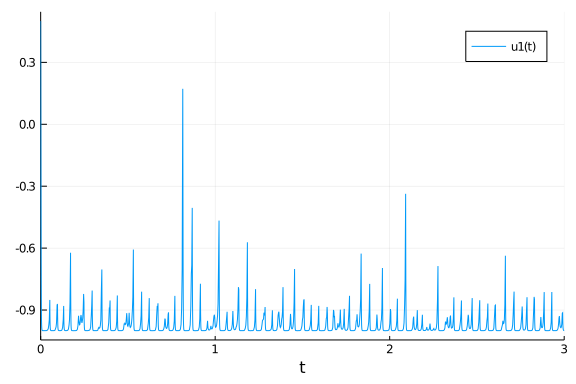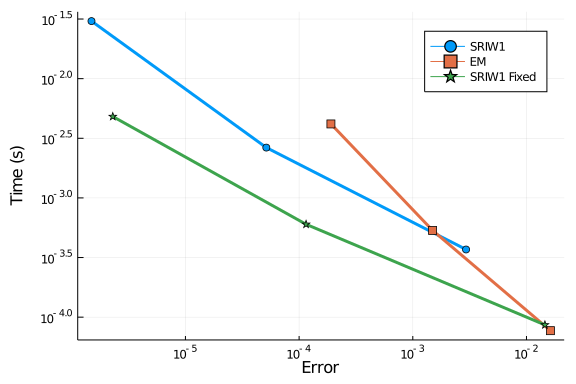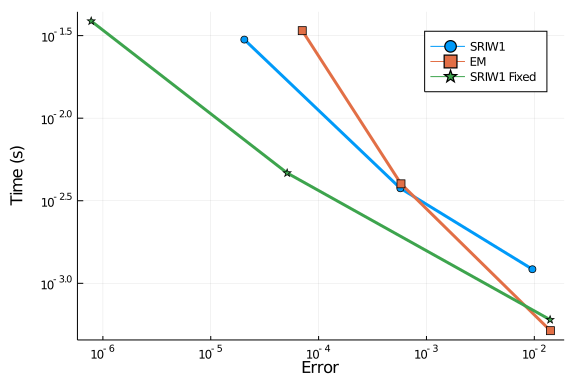##### Chris Rackauckas

In this notebook we will explore the quadratic stiffness problem. References:

The composite Euler method for stiff stochastic differential equations

Kevin Burrage, Tianhai Tian

And

S-ROCK: CHEBYSHEV METHODS FOR STIFF STOCHASTIC DIFFERENTIAL EQUATIONS

ASSYR ABDULLE AND STEPHANE CIRILLI

This is a scalar SDE with two arguments. The first controls the deterministic stiffness and the later controls the diffusion stiffness.

using DiffEqProblemLibrary, StochasticDiffEq, DiffEqDevTools
using DiffEqProblemLibrary.SDEProblemLibrary: importsdeproblems; importsdeproblems()
using Plots; gr()
const N = 10

10

prob = remake(prob_sde_stiffquadito,p=(50.0,1.0))
sol = solve(prob,SRIW1())
plot(sol)prob = remake(prob_sde_stiffquadito,p=(500.0,1.0))
sol = solve(prob,SRIW1())
plot(sol)## Top dts

Let's first determine the maximum dts which are allowed. Anything higher is mostly unstable.

### Deterministic Stiffness Mild

prob = remake(prob_sde_stiffquadito,p=(50.0,1.0))
@time sol = solve(prob,SRIW1())

0.000149 seconds (1.82 k allocations: 71.609 KiB)

@time sol = solve(prob,SRIW1(),adaptive=false,dt=0.01)

0.179881 seconds (555.49 k allocations: 29.716 MiB, 10.63% gc time)

@time sol = solve(prob,ImplicitRKMil(),dt=0.005)

11.318100 seconds (25.57 M allocations: 7.089 GiB, 7.70% gc time)

@time sol = solve(prob,EM(),dt=0.01);

10.489591 seconds (22.45 M allocations: 6.932 GiB, 8.27% gc time)
retcode: Success
Interpolation: 1st order linear
t: 302-element Array{Float64,1}:
0.0
0.01
0.02
0.03
0.04
0.05
0.060000000000000005
0.07
0.08
0.09
⋮
2.9299999999999815
2.9399999999999813
2.949999999999981
2.959999999999981
2.9699999999999807
2.9799999999999804
2.9899999999999802
2.99999999999998
3.0
u: 302-element Array{Float64,1}:
0.5
0.13396570587790263
-0.2877533207819682
-0.6906409774455424
-0.9743764006275005
-1.0030831461101295
-1.00006474984873
-0.9999947620238386
-1.000000256944763
-0.9999999582774294
⋮
-1.0
-1.0
-1.0
-1.0
-1.0
-1.0
-1.0
-1.0
-1.0


### Deterministic Stiffness High

prob = remake(prob_sde_stiffquadito,p=(500.0,1.0))
@time sol = solve(prob,SRIW1())

0.000894 seconds (13.91 k allocations: 537.812 KiB)

@time sol = solve(prob,SRIW1(),adaptive=false,dt=0.002)

0.000583 seconds (10.60 k allocations: 438.906 KiB)

@time sol = solve(prob,ImplicitRKMil(),dt=0.001)

0.000066 seconds (457 allocations: 18.828 KiB)

@time sol = solve(prob,EM(),dt=0.002);

0.000524 seconds (7.59 k allocations: 359.406 KiB)
retcode: Success
Interpolation: 1st order linear
t: 1502-element Array{Float64,1}:
0.0
0.002
0.004
0.006
0.008
0.01
0.012
0.014
0.016
0.018000000000000002
⋮
2.9859999999998927
2.9879999999998925
2.9899999999998923
2.991999999999892
2.993999999999892
2.9959999999998916
2.9979999999998914
2.999999999999891
3.0
u: 1502-element Array{Float64,1}:
0.5
-0.24758498154895137
-1.1174008836737759
-0.8689850517530578
-1.10883889092748
-0.8859367575119022
-1.0807779204418193
-0.9078534903011535
-1.075990006315383
-0.9231031131175731
⋮
-0.9999999588620538
-1.0000000387882142
-0.9999999615688911
-1.0000000375969333
-0.9999999603664674
-1.0000000440192396
-0.9999999605034843
-1.0000000367007533
-1.0000000367007993


### Mixed Stiffness

prob = remake(prob_sde_stiffquadito,p=(5000.0,70.0))
@time sol = solve(prob,SRIW1(),dt=0.0001)

0.089729 seconds (417.72 k allocations: 23.278 MiB)

@time sol = solve(prob,SRIW1(),adaptive=false,dt=0.00001)

0.135112 seconds (2.10 M allocations: 70.361 MiB, 14.92% gc time)

@time sol = solve(prob,ImplicitRKMil(),dt=0.00001)

0.270491 seconds (1.00 M allocations: 61.394 MiB)

@time sol = solve(prob,EM(),dt=0.00001);

0.112811 seconds (1.50 M allocations: 56.205 MiB, 11.37% gc time)
retcode: Success
Interpolation: 1st order linear
t: 300001-element Array{Float64,1}:
0.0
1.0e-5
2.0e-5
3.0000000000000004e-5
4.0e-5
5.0e-5
6.0e-5
7.000000000000001e-5
8.0e-5
9.0e-5
⋮
2.9999200000111856
2.9999300000111857
2.9999400000111858
2.999950000011186
2.999960000011186
2.999970000011186
2.999980000011186
2.999990000011186
3.0
u: 300001-element Array{Float64,1}:
0.5
0.4599641306769577
0.3599996341364714
0.6311092879078233
0.8303248474493431
0.7369579270353366
0.7136566685173701
0.7594703464273537
0.580947370183962
0.3261632407453182
⋮
-1.0
-1.0
-1.0
-1.0
-1.0
-1.0
-1.0
-1.0
-1.0


Notice that in this problem, the stiffness in the noise term still prevents the semi-implicit integrator to do well. In that case, the advantage of implicitness does not take effect, and thus explicit methods do well. When we don't care about the error, Euler-Maruyama is fastest. When there's mixed stiffness, the adaptive algorithm is unstable.

## Work-Precision Diagrams

prob = remake(prob_sde_stiffquadito,p=(50.0,1.0))

reltols = 1.0 ./ 10.0 .^ (3:5)
abstols = reltols#[0.0 for i in eachindex(reltols)]
setups = [Dict(:alg=>SRIW1()),
Dict(:alg=>EM(),:dts=>1.0./8.0.^((1:length(reltols)) .+ 1)),
]
names = ["SRIW1","EM","SRIW1 Fixed"] #"RKMil",
wp = WorkPrecisionSet(prob,abstols,reltols,setups;numruns=N,names=names,error_estimate=:l2)
plot(wp)prob = remake(prob_sde_stiffquadito,p=(500.0,1.0))

reltols = 1.0 ./ 10.0 .^ (3:5)
abstols = reltols#[0.0 for i in eachindex(reltols)]
setups = [Dict(:alg=>SRIW1()),
Dict(:alg=>EM(),:dts=>1.0./8.0.^((1:length(reltols)) .+ 2)),
]
names = ["SRIW1","EM","SRIW1 Fixed"] #"RKMil",
wp = WorkPrecisionSet(prob,abstols,reltols,setups;numruns=N,names=names,error_estimate=:l2,print_names=true)
plot(wp)## Conclusion

Noise stiffness is tough. Right now the best solution is to run an explicit integrator with a low enough dt. Adaptivity does have a cost in this case, likely due to memory management.

using DiffEqBenchmarks
DiffEqBenchmarks.bench_footer(WEAVE_ARGS[:folder],WEAVE_ARGS[:file])


## Appendix

These benchmarks are a part of the DiffEqBenchmarks.jl repository, found at: https://github.com/JuliaDiffEq/DiffEqBenchmarks.jl

To locally run this tutorial, do the following commands:

using DiffEqBenchmarks
DiffEqBenchmarks.weave_file("StiffSDE","QuadraticStiffness.jmd")

Computer Information:

Julia Version 1.4.2
Commit 44fa15b150* (2020-05-23 18:35 UTC)
Platform Info:
OS: Linux (x86_64-pc-linux-gnu)
CPU: Intel(R) Core(TM) i7-9700K CPU @ 3.60GHz
WORD_SIZE: 64
LIBM: libopenlibm
LLVM: libLLVM-8.0.1 (ORCJIT, skylake)
Environment:
JULIA_DEPOT_PATH = /builds/JuliaGPU/DiffEqBenchmarks.jl/.julia
JULIA_CUDA_MEMORY_LIMIT = 2147483648
JULIA_PROJECT = @.

Status: /builds/JuliaGPU/DiffEqBenchmarks.jl/benchmarks/StiffSDE/Project.toml
[10745b16-79ce-11e8-11f9-7d13ad32a3b2] Statistics